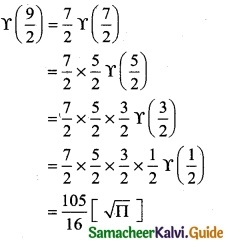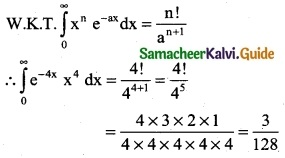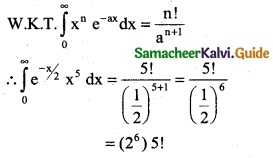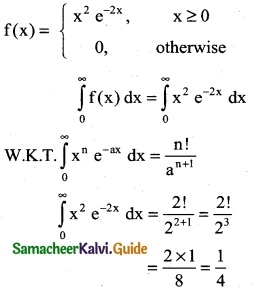Tamilnadu State Board New Syllabus Samacheer Kalvi 12th Business Maths Guide Pdf Chapter 2 Integral Calculus I Ex 2.10 Text Book Back Questions and Answers, Notes.

## Tamilnadu Samacheer Kalvi 12th Business Maths Solutions Chapter 2 Integral Calculus I Ex 2.10

Evaluate the following:

Question 1.
(i) $$\Upsilon$$ (4)
Solution:
Γ(4) = Γ(3 + 1) = 3! = 6(ii) $$\Upsilon$$ ($$\frac { 9 }{2}$$)
Solution:(iii) $$\int_{0}^{∞}$$ e-mx x6 dx
Solution:
W.K.T $$\int_{0}^{∞}$$ xⁿ e-ax dx = $$\frac { n! }{a^{n+1}}$$
∴ $$\int_{0}^{∞}$$ e-mx x6 dx = $$\frac { 6! }{3^{6+1}}$$ = $$\frac { 6! }{m^7}$$(iv) $$\int_{0}^{∞}$$ e-4x x4 dx
Solution:(v) $$\int_{0}^{∞}$$ e-x/2 x5 dx
Solution:Question 2.
If f(x) = $$\left\{\begin{array}{l} x^{2} e^{-2 x}, x \geq 0 \\ 0, \text { otherwise } \end{array}\right.$$, then evaluate $$\int_{0}^{∞}$$ f(x) dx
Solution:
Given